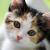# java 多线程的问题

package com.cbd.zhixiang;

{
public static void main(String[] args)
{
Ticket t = new Ticket();
}
}

class Ticket  implements Runnable
{
private int count = 100;

Object obj = new Object();
public void run()
{
synchronized (obj)
{

while (count > 0)

try
{
} catch (InterruptedException e)
{
e.printStackTrace();
}
+ count--);
}
}
}
}

0```class Ticket  implements Runnable
{
private int count = 100;

public void run()
{
Object obj = new Object();
synchronized (obj)
{

while (count > 0)
{
try
{
} catch (InterruptedException e)
{
e.printStackTrace();
}
+ count--);
}```
00new Thread(t).start();三个线程共用的是同一个对象，那么Object obj = new Object();这么一来，三个线程共用一把锁了。@古代瑞兽 这不是休眠的问题，那我问题你加这个休眠意义何在呢？@古代瑞兽 @j&a如果去掉休眠，是不是就不存在问题了？
0```public class Ticket {
private int total;

public Ticket(int total) {
super();
this.total = total;
}

public int getTotal() {
}
}```

```import java.util.concurrent.ExecutorService;
import java.util.concurrent.Executors;
import java.util.concurrent.atomic.AtomicReference;

public class BuyTicket implements Runnable {
private int got;
private AtomicReference<Ticket> ticket;
private boolean stop;
private String name;
public BuyTicket(AtomicReference<Ticket> ticket, String name) {
super();
this.ticket = ticket;
this.name = name;
}

@Override
public void run() {
try {
Ticket current = null;
Ticket next = null;
do {
current = ticket.get();
if (current.getTotal() <= 0) {
stop = true;
}
next = new Ticket(current.getTotal() - 1);
} while (!this.ticket.compareAndSet(current, next));
this.got++;
} catch (Exception e) {
stop = true;
}
}
+ this.got + " tickets");
}

public int getGot() {
return got;
}

public void setGot(int got) {
this.got = got;
}

public static void main(String args[]) {
int total = 100000;
AtomicReference<Ticket> atomic = new AtomicReference<Ticket>(new Ticket(total));
System.out.println("Start ! There are " + atomic.get().getTotal() + " tickets left.");